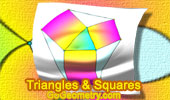# Geometry: Triangle and Squares, Theorems and Problems 2The Pythagorean Curiosity Triangles and squares, fifteen conclusions. Albert Einstein and the Pythagorean theorem. A preteen Albert Einstein devised a new proof of the Pythagorean theorem. Proposed Problem 305. Square, Triangles, Angles, Sides. Kurschak's Tile and Theorem. Jozsef Kurschak (Hungary, 1864-1933) An elegant and a purely geometric way of finding the area of a regular dodecagon. A square with an equilateral triangle drawn inwards on each side. Euclid's Elements, Book X, Lemma for Proposition 33 One page visual illustration. Archimedes' Book of Lemmas: Proposition 7 Square and inscribed and circumscribed Circles Proposed Problem 273. Triangle, Perpendiculars, Area of Squares. Proposed Problem 276. Square, 90 degree Arcs, Circle, Radius. Proposed Problem 265. Right Triangle, Pythagorean Theorem. Proposed Problem 244. Square, Diagonals, Centers, Distance. Proposed Problem 239. Square, Midpoints, Lines, Congruence, Pythagoras. Proposed Problem 238. Square, Midpoints, Lines, Congruence. Archimedes Arbelos and Square 2. Dynamic Geometry Software. Step-by-Step construction, Manipulation, and animation. Archimedes Arbelos and Square 1. Dynamic Geometry Software. Step-by-Step construction, Manipulation, and animation. Proposed Problem 189. Squares, Distances. Proposed Problem 188. Square, Diagonal, 45 Degrees Angle. Proposed Problem 179. Rhombus, Square, Diagonal, Angle. Proposed Problem 112. Area of square and triangle. Inca Skull Surgery Inca Skull Surgeons Were "Highly Skilled," Study Finds. Trepanation = square hole in the skull. Huancayo Square the Wankas Ayacucho Square (Plaza de Armas) Ayacucho video Triangle and Squares Theorem 1: with Interactive Geometry Software Step-by-Step construction, Manipulation, and animation. Geometry Problem 1344, Bottema's Theorem: Triangle and Squares with Interactive Geometry Software. Interactive Hinged tessellation. Square tessellation - Dynamic Geometry. Please be patient while the applet loads on your computer. This Java applet requires Java 1.3 or higher. Van Aubel's Theorem. Quadrilateral with Squares. Proof with animation. Triangle and Squares 21 See also: Triangle and Squares 19 Triangle and Squares 18  Sangaku Problem

Home | Sitemap | SearchGeometry | Post a comment | Email
Last updated: Mar 6, 2016